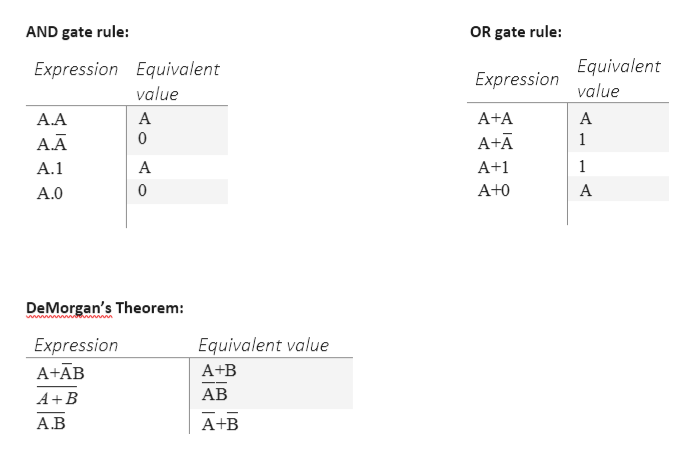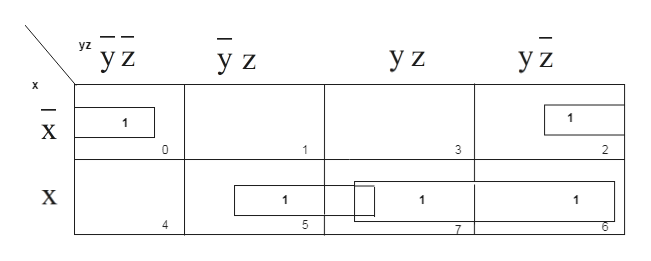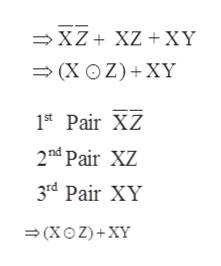PLEASE EXPLAIN ALL STEPS (including how k-map formation is formed): simplify the boolean equations: xz + (xy + ~z) ~xyz + yz + x~y

Question

PLEASE EXPLAIN ALL STEPS (including how k-map formation is formed):

simplify the boolean equations:

1. xz + (xy + ~z)
2. ~xyz + yz + x~y
Step 1

Some of the simple rules follows to simplify Boolean expression:help_outlineImage TranscriptioncloseAND gate rule: OR gate rule: Expression Equivalent value Expression Equivalent value А A+A А А.А 0 A+A 1 A.A 1 A+1 A.1 0 A+0 A А.О DeMorgan's Theorem: Expression Equivalent value A+AB A+B АВ AB А.В A+B fullscreen
Step 2

Simplify the Boolean equations: xz + (xy + ~z)

For simplifying the Boolean expression, use Karnaugh Map and follow the below steps-

1. a) Fill 1 at decimal equivalent number 0,2,5,6,7 as per the given question accordingly in the K-map.
2. b) Then pairing will be done in the K-map.

c)There will be three pairs formed in this K -map as shown below –help_outlineImage Transcriptionclose_ yz УZ УZ 1 1 X 0 1 3 2 X 1 1 7 IN | N fullscreen
Step 3

The final expression i...help_outlineImage TranscriptioncloseXZ XZ XY (X OZ)XY 1t Pair XZ 2nd Pair XZ 3rd Pair XY (XO Z)+XY fullscreen

Want to see the full answer?

See Solution

Want to see this answer and more?

Our solutions are written by experts, many with advanced degrees, and available 24/7

See Solution
Tagged in

Computer Architecture